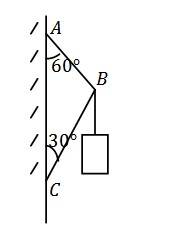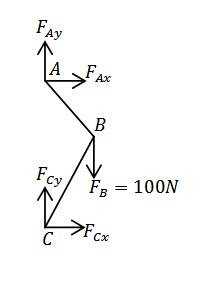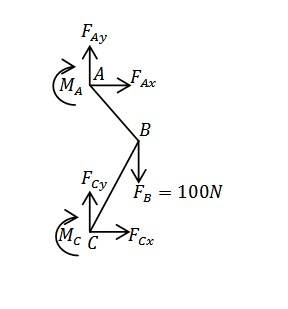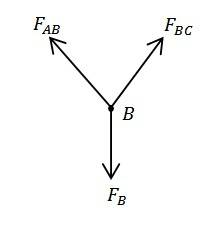# Determine the magnitude and direction of forces

## Homework StatementThe figure shows a frame with a 100N object hanging on it. Determine the magnitude and direction of forces exerted on AB and BC respectively.

## Homework Equations

∑Fx=0, ∑Fy=0, ∑M=0

## The Attempt at a Solution

I believe this question is unsolvable. The free body diagram of the frame is as follows:There are 4 unknowns in the diagram but we can only write down 3 equations. Hence, there is no way we can solve this problem.

Is my reasoning correct?

I apologize for my mistake. The force diagram should be like this:Thus, we have 6 unknowns and 3 equations. I deem it unsolvable.

SteamKing
Staff Emeritus
Homework Helper
Your FBDs are not exactly correct. You aren't given any information about member BC or how it is fixed to the wall, so assuming that there is a moment at point C is probably incorrect. Similarly, AB is probably a continuation of the same line which supports the 100N object, and it would not be capable of supporting a moment at point A.

I think if you analyze the forces acting at point B, you will get a better handle on this problem. Once you know what is going on at B, then you can figure out what happens at A and C to support the load and keep everything in equilibrium.

Your FBDs are not exactly correct. You aren't given any information about member BC or how it is fixed to the wall, so assuming that there is a moment at point C is probably incorrect. Similarly, AB is probably a continuation of the same line which supports the 100N object, and it would not be capable of supporting a moment at point A.

I think if you analyze the forces acting at point B, you will get a better handle on this problem. Once you know what is going on at B, then you can figure out what happens at A and C to support the load and keep everything in equilibrium.

Yes, I wasn't given any information about how the frame is fixed to the wall. So I assumed it was fixed support that allows reactive moment.

Anyway, I don't understand how we can analyze "the forces acting at a point". Is it true that when we analyze a problem in statics, we must first define the system? In my FBD, I define the system as the beam.

So my question is, when we analyze the forces acting at a point, say point B, what is the system?

SteamKing
Staff Emeritus
Homework Helper
If point B is not moving, then the sum of the forces acting at point be B must be zero. This is Newton's Second Law of Motion. If you draw a Free Body Diagram which isolates Point B, knowing the orientation of the line AB and the strut BC should give you the direction in which these forces act to support the 100 N weight.

Thanks SteamKing. It seems to me that you are saying point B is the system. Am I right?

The FBD that you described should look like this:Yes, by using the above FBD, things become rather easy. In fact what you explained was similar to the explanation given by the textbook. You mentioned that the direction of forces acting on B can be determined by the orientation of line AB and line BC. Why is that so?

SteamKing
Staff Emeritus
Homework Helper
You mentioned that the direction of forces acting on B can be determined by the orientation of line AB and line BC. Why is that so?

The line supporting the weight, which also runs between AB, can support only a tension force. The direction of this force must be coincident with AB, otherwise a moment will develop, which the line cannot support.

Similarly, if we assume that the strut BC is not built into the wall, no moment can be developed at Point C. Therefore, a compressive force will develop in the strut, due to the suspended weight, and this compressive force must also be aligned with BC.

The line supporting the weight, which also runs between AB, can support only a tension force.

If AB is a rope, well,then I agree that only tension forces can exist thus the direction of force should be along AB.Since the question already mentioned that it is a beam, I suppose the structure can support shear force. Right? If so, why must the force be along AB?

a compressive force will develop in the strut, due to the suspended weight
What are the subject and the object of the compressive force?

SteamKing
Staff Emeritus
Homework Helper
OK, let's assume that AB and BC are some sort of framework from which the 100 N load is suspended. Based on the information in the OP, let's assume the frame remains static. We are not given any information about how the frame is attached at points A and C, so for simplicity, let's also assume the frame is pinned at these two points.

We can still write equations of static equilibrium for the frame about either point A or C, using your first FBD. We make no assumptions here initially about whether the forces in the frame are tensile or compressive. The sum of the forces in the horizontal and vertical directions must still sum to zero in order for the frame to remain stationary, and since the load is vertical, that means that FAx + FCx = 0. We can see here that the direction of the horizontal components of the forces in the frame members must act in opposite directions for the frame to remain stationary, so it is reasonable also to conclude that one member is in tension and the other is in compression.

If we were to release the frame at Point A by removing the pin, FAx would have to be directed toward the wall in order to keep the frame static, so this also implies, given the equilibrium of the horizontal forces, that FCx must act away from the wall, in the opposite direction of FAx.

We can do a similar qualitative analysis of the forces acting in the vertical direction, FAy and FCy, but here we must also consider the moment of the 100 N load which is created by Point B being offset from the wall.

Exactly.

By using the first FBD, we have 4 unknowns and 3 equations. Thus, this problem is unsolvable, isn't it?

As for the third FBD, which analyzes forces acting on Point B, I believe it is flawed because Fab may not act along line AB, and Fbc may not act along line BC. However, teachers and textbooks have been using the third FBD to approach the problem for a long time.

SteamKing
Staff Emeritus
Homework Helper
If you are just going to throw up your hands without trying to analyze the problem fully, I can't help you any further. You can make all sorts of assumptions about how forces are aligned in this problem, but most of them won't lead you to a solution.

Which part of my replies gave you, Sir, an impression that I throw up my hands? I am happy to know that. I spent like 10 hrs on this problem before I posted this thread.

Anyway, have you solved the problem yourself, Sir?

You mentioned "FAx + FCx = 0", and your analysis indicated that FAx and FCx must be opposite in direction. Well, I have done this part of the analysis and that's why I said "exactly" in my previous post. I even went further to discover that one can never determine the magnitude of FAx and FCx unless the deformation of the beam at point A and point C are known.

Please solve the problem and see for yourself, Sir.

"If A, B, C are pin connected, the system is a truss and members AB and BC are two force members which can only take tension or compression."

A professor whom I asked yesterday made everything clear with the above sentence.

Thank you SteamKing for all your help. I really appreciate that.